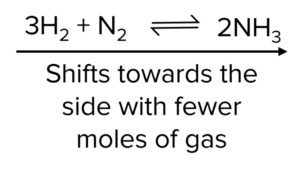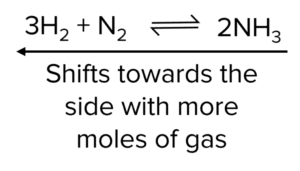# Equilibria & Le Chateliers Principle

A LevelAS LevelAQA

## Equilibria and Le Châtelier’s Principle

Chemical equilibrium is a fascinating phenomenon in chemical reactions. In these systems, both forwards and backwards reactions happen at the same time. The positions of equilibrium (how far towards products or reactants a reaction is) can be greatly affected by the conditions under which the reactions takes place. How equilibrium responds to external conditions is governed by Le Châteliers principle.

## Equilibrium

Many chemical reactions are reversible. This means that reaction can take place in both the forwards and backwards direction. For example, the reaction between hydrogen and iodine can take place both directions:

\begin{aligned}\text{H}_2+\text{I}_2&\rarr2\text{HI}\\2\text{HI}&\rarr\text{H}_2+\text{I}_2\end{aligned}

At equilibrium, both reactions will happen simultaneously with equal rates of reaction. This is known as dynamic equilibrium. We represent this in the chemical equation by using two parallel arrows, pointing in the opposite direction:

$\text{H}_2+\text{I}_2\rightleftharpoons 2\text{HI}$

In reactions that are taking place under dynamic equilibrium, the concentrations of both reactants and products will remain constant. This is because they are being both formed and used up at the same rate.

A LevelAS LevelAQA

## Le Châtelier’s Principle

Le Châtelier’s principle governs the responses of chemical equilibria to changes in their conditions.

Le Châtelier’s Principle: Equilibria will change their position to counteract any change in their conditions.

What this means in practice is that, in response to an external change, an equilibrium reaction will move further towards which ever side of the reaction best opposes this change. For example, the temperature of an equilibrium redaction takes place, the equilibrium will shift to the endothermic side of the reaction (i.e. whatever side produces the lowest temperature). This shift will lead to an increase in either the products or the reactants of the reaction.

Le Châtelier’s principle is extremely useful in industrial chemistry as it allows us to manipulate reactions to produce as much product as possible.

A LevelAS LevelAQA

## Effects of Pressure

Increase in Pressure

If the pressure of a system is increased, the equilibrium opposes the change by shifting to the side which produces the fewest moles of gas to reduce the pressure.Decrease in Pressure

If the pressure of a system is decreased, the equilibrium opposes the change by shifting to the side which produces the most moles of gas to increase the pressure.In some cases, the moles of gas are equal on both sides. If these cases changes in pressure will have no effect on the position of the equilibrium.

Industries will sometimes induce high pressures to increase the yield of a product. However this is only possible up to a certain pressure. Higher pressures are more expensive to maintain due to the need to ensure safety, as well as to supply the energy required to induce a high pressure.

A LevelAS LevelAQA

## Effects of Temperature

Increase in Temperature

If the temperature of a system is increased, the equilibrium shifts in the endothermic direction to oppose the change and reduce the temperature by absorbing heat.

Decrease in Temperature

If the temperature of a system is decreased, the equilibrium shifts in the exothermic direction to oppose the change and increase the temperature by giving out heat.

For example, take the formation of methanol:

$\text{CO}_{(g)}+2\text{H}_{2 (g)}\rightleftharpoons\text{CH}_3\text{OH}_{(g)} [\Delta\text{H}=-90\text{ kJ mol}^{-1}]$

In the above equilibrium, the forward reaction is exothermic. Therefore if temperature is increased the backwards reaction would be favoured since it is endothermic.

A LevelAS LevelAQA

## Effects of Concentration

$\text{H}_{2\left(\text{g}\right)}+\text{I}_{2\left(\text{g}\right)}\leftrightarrows2\text{HI}_{\left(\text{g}\right)}$

Increasing the concentration of $\text{I}_2$ will cause the equilibrium to shift to the right to oppose the change by removing the excess and decrease the concentration of $\text{I}_2$ ions. This would give a higher yield of $\text{HI}$. Decreasing the concentration of $\text{I}_2$ would cause a shift to the left to replace the loss. This reduces the yield of $\text{HI}$

A LevelAS LevelAQA

## Effects of Catalysts

Catalysts do not affect the position of equilibrium as they speed up the rate of the reaction of both the forward and backward reaction by the same amount. Catalysts will however cause the system to reach equilibrium more quickly

A LevelAS LevelAQA

## Industrial Processes

Le Châtelier’s principle is used in industry to maximise yield. You need to know about how Le Châtelier’s principle is applied to the following industrial processes. Though you will not need to know the values of the enthalpy changes for these reactions, you do need to know the chemical equations involved and the effects that changing conditions will have on them.

Contact Process

\begin{aligned}\text{Stage 1: S}_{(s)}+\text{O}_{2 (g)}&\rarr\text{SO}_{2 (g)}\\\text{Stage 2: SO}_{2 (g)}+\frac{1}{2}\text{O}_{2 (g)}&\rightleftharpoons\text{SO}_{3 (g)}\end{aligned}

$\Delta\text{H}=-97 \text{ kJ mol}^{-1}$

Conditions

• Temperature: $450\degree\text{C}$
• Pressure: $1-2\text{ atm}$
• Catalyst: $\text{V}_2\text{O}_5$

Temperature: Stage 2 of the contact process is exothermic so a low temperature is needed to increase the yield. However, a low temperature would cause a slow rate so a compromise is made of $450\degree\text{C}$.

Pressure: Having a high pressure would lead to high energy costs for marginal gains. This is because the effect of high pressure on yield is only slightly better.

Haber Process

$\text{N}_{2\left(\text{g}\right)}+3\text{H}_{2\left(\text{g}\right)}\rightleftharpoons2\text{NH}_{3\left(\text{g}\right)}$

$\Delta\text{H}=-92\text{ kJ mol}^{-1}$

Conditions

• Temperature: $450\degree\text{C}$
• Pressure: $200-1000\text{ atm}$
• Catalyst: Iron

Temperature: The Haber Process is exothermic so a low temperature is needed to increase the yield. However, a low temperature would cause a slow rate so a compromise is made of 450℃.

Pressure: Having a high pressure would lead to a higher yield as there are fewer moles of gas on the right. Therefore, high pressure is used. The pressure used is not too high because it would be expensive to maintain.

Hydration of Ethene

$\text{C}_2\text{H}_{4\left(\text{g}\right)}+\text{H}_2\text{O}_{\left(\text{g}\right)}\rightleftharpoons\text{CH}_3\text{CH}_2\text{OH}_{\left(\text{l}\right)}$

Conditions

• Temperature: $300\degree\text{C}$
• Pressure: $60-70\text{ atm}$
• Catalyst: Concentrated Phosphoric Acid

Temperature: The hydration of ethene is exothermic so a low temperature is needed to increase the yield. However, a low temperature would cause a slow rate so a compromise is made of $300\degree\text{C}$.

Pressure: Having a high pressure would lead to a higher yield as there are fewer moles on the right so a high pressure is used. However, too high a pressure would lead to ethene being polymerised so a compromise of $60-70\text{ atm}$ is made.

Production of Methanol

$\text{CO}_{\left(\text{g}\right)}+2\text{H}_{2\left(\text{g}\right)}\rightleftharpoons\text{CH}_3\text{OH}_{\left(\text{g}\right)}$

Conditions

• Temperature: $400\degree\text{C}$
• Pressure: $50\text{ atm}$
• Catalyst: Chromium and zinc oxides

Temperature: The production of methanol is exothermic so a low temperature is needed to increase the yield. However, a low temperature would cause a slow rate so a compromise is made of $400\degree\text{C}$.

Pressure: Having a high pressure would lead to a higher yield as there are fewer moles on the right so a high pressure is used. The pressure used is not too high because it would be expensive to maintain.

Note: In industry, unreacted reactants are often recycled back into the reactor to improve the yield.

A LevelAS LevelAQA

## Equilibria & Le Chateliers Principle Example Questions

• The equilibrium will shift to oppose the change and reduce the pressure.
• So will shift to the left since there are fewer moles of gas; there is $1$ mole on the left and $2$ moles on the right.
• Gives a lower yield of $\text{NO}_2$
• High pressure = Equilibrium shifts to the left/ lower yield
• High pressure = Increased rate
• Increased pressure does this because there are more moles of gas on the right.
• High temp = Equilibrium shifts to the right/ yield is greater
• High temp = Increased rate
• High temp does this because the reaction is endothermic.

Temperature

• The reaction is exothermic
• A high temperature lowers the yield.
• But a higher temperature leads to a higher rate of reaction

Pressure

• $4$ moles of gaseous reactants form $2$ moles of gaseous product
• A high pressure increases the yield.
• But a higher pressure generation and equipment is expensive.

## You May Also Like...### MME Learning Portal

Online exams, practice questions and revision videos for every GCSE level 9-1 topic! No fees, no trial period, just totally free access to the UK’s best GCSE maths revision platform.

£0.00